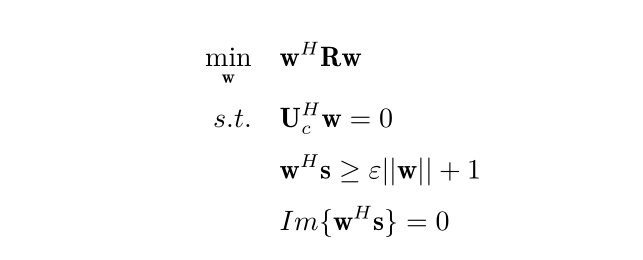# How can I solve this convex QC problem using CVX?R is a positive Hermitian matrix, and U_c is a full column matrix and \mathbf{w}\in\mathbb{C}^N is a column vector, and Im\{\cdot\} denotes the image part of the complex number.
I don’t known how to solve this optimization using CVX, can you help me.
Thank you very much.

1 Like

Have you read the CVX Users’ Guide http://cvxr.com/cvx/doc/ ? This problem can be entered essentially directly as shown into CVX. (H is ' and Im is imag)

1 Like

but something wrong in my programming. can you help me?

1 Like

Perhaps R  is not exactly Hermitian, with the consequence that there is a roundoff level imaginary term in the quadratic form. You could try Hermitianizing it.
w'*(0.5*(R+R'))*w <= const

If that still produces the same error message, perhaps R isn’t actually Hermitian Semidefinite. What is the value of min(eig(R+R')) ?

1 Like

thank you！
I will try again.
how can I transform this problem to SDP?
can you help me?

1 Like

The problem will be solved by CVX as an SOCP. Any SOCP can be written as an SDP. But it is better to solve it as an SOCP. Indeed, if you convert and enter this as an SDP in CVX, CVX will automatically transform it to an SOCP before sending it to the solver.

You need to fix R, as I described previously.

1 Like

hello ,there are something wrong in my programming, can you help me ?
Thank you very much.

1 Like

CVX only allows inequalities in which both sides are real.

Why does
w'*s >= epsilon*norm(w)+1
make sense? The left-hand side is complex, and the right-hand side is real.

Do you perhaps want
real(w'*s) >= epsilon*norm(w)+1

I have no idea whether that makes sense. For purposes of this forum, it’s your model, even if you got it from a paper or book, so you tell us what it’s supposed to be.

1 Like

as you can see, in my formulation, imag(w’*s)==0, which aims to let the image part of w’*s is zero, therefore, w’*s is a real number. i think real(w’s) >= epsilonnorm(w)+1 is right.
thank you very much.

1 Like

Yes, I believe so. .

1 Like